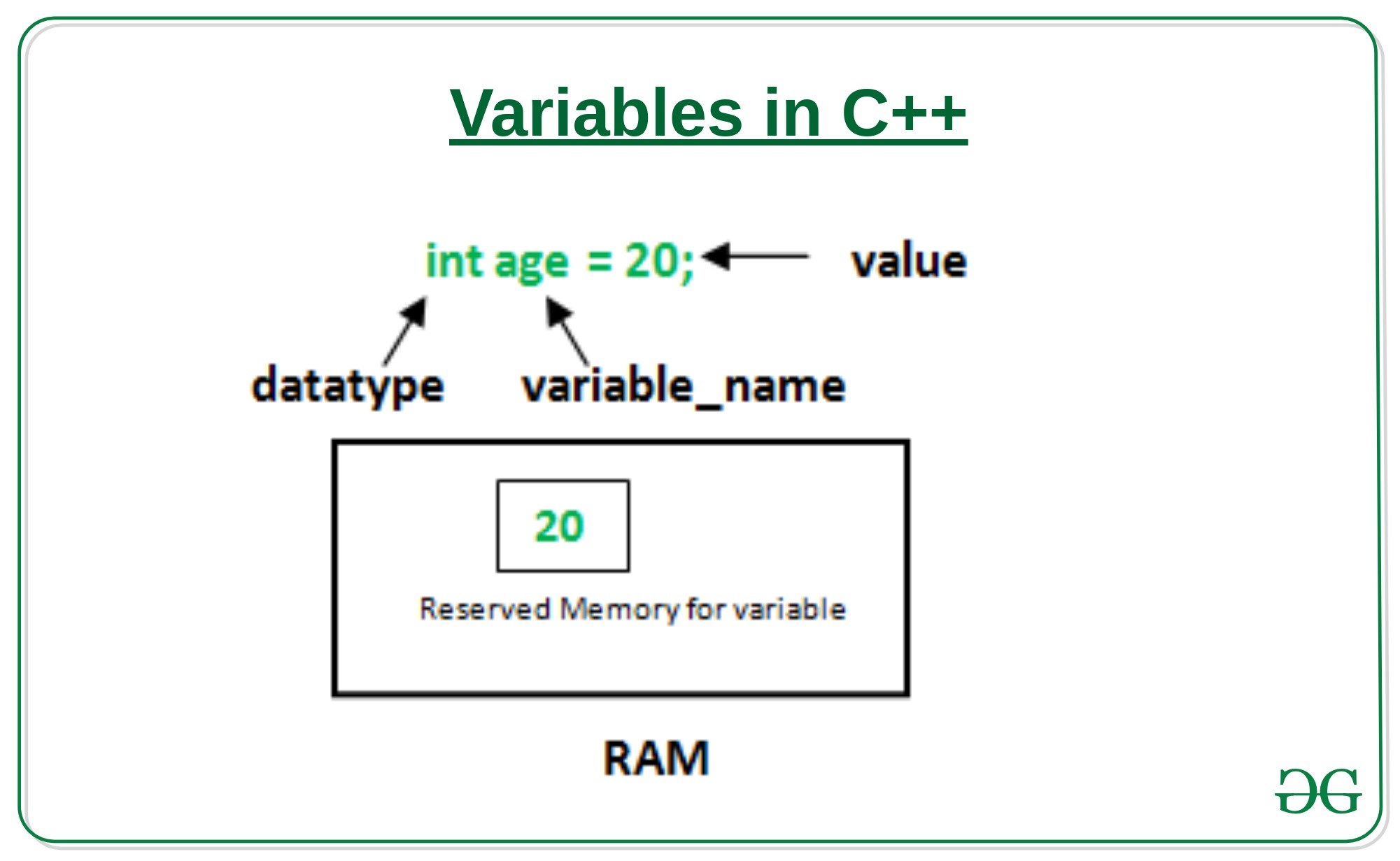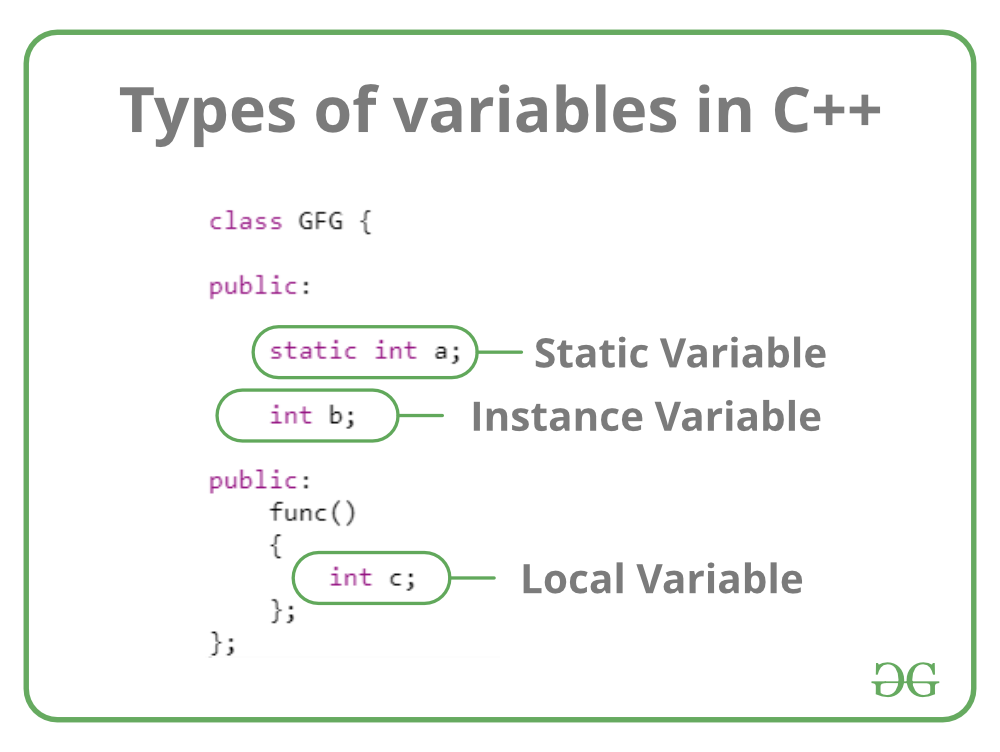# Variables in C++

A variable is a name given to a memory location. It is the basic unit of storage in a program.

• The value stored in a variable can be changed during program execution.
• A variable is only a name given to a memory location, all the operations done on the variable effects that memory location.
• In C++, all the variables must be declared before use.

How to declare variables?

A typical variable declaration is of the form:

```// Declaring a single variable
type variable_name;

// Declaring multiple variables:
type variable1_name, variable2_name, variable3_name;
```

A variable name can consist of alphabets (both upper and lower case), numbers and the underscore ‘_’ character. However, the name must not start with a number.In the above diagram,

datatype: Type of data that can be stored in this variable.
variable_name: Name given to the variable.
value: It is the initial value stored in the variable.

Examples:

```// Declaring float variable
float simpleInterest;

// Declaring integer variable
int time, speed;

// Declaring character variable
char var;
```

Difference between variable declaration and definition

The variable declaration refers to the part where a variable is first declared or introduced before its first use. A variable definition is a part where the variable is assigned a memory location and a value. Most of the times, variable declaration and definition are done together.

See the following C++ program for better clarification:

 `#include ` `using` `namespace` `std; ` ` `  `int` `main() ` `{ ` `    ``// declaration and definition ` `    ``// of variable 'a123' ` `    ``char` `a123 = ``'a'``; ` ` `  `    ``// This is also both declaration and definition ` `    ``// as 'b' is allocated memory and ` `    ``// assigned some garbage value. ` `    ``float` `b; ` ` `  `    ``// multiple declarations and definitions ` `    ``int` `_c, _d45, e; ` ` `  `    ``// Let us print a variable ` `    ``cout << a123 << endl; ` ` `  `    ``return` `0; ` `} `

Output:

```a
```

Types of variables

There are three types of variables based on the scope of variables in C++:

• Local Variables
• Instance Variables
• Static VariablesLet us now learn about each one of these variables in detail.

1. Local Variables: A variable defined within a block or method or constructor is called local variable.

• These variable are created when the block in entered or the function is called and destroyed after exiting from the block or when the call returns from the function.
• The scope of these variables exists only within the block in which the variable is declared. i.e. we can access these variable only within that block.
• Initilisation of Local Variable is Mandatory.

Sample Program 1:

 `// C++ program to demonstrate Local variables ` ` `  `#include ` `using` `namespace` `std; ` ` `  `void` `StudentAge() ` `{ ` `    ``// local variable age ` `    ``int` `age = 0; ` `    ``age = age + 5; ` `    ``cout << ``"Student age is : "` `<< age; ` `} ` ` `  `// Driver code ` `int` `main() ` `{ ` `    ``StudentAge(); ` `} `

Output:

```Student age is : 5
```

In the above program, the variable age is a local variable to the function StudentAge(). If we use the variable age outside StudentAge() function, the compiler will produce an error as shown in below program.

Sample Program 2:

 `// C++ program to demonstrate Local variables ` ` `  `#include ` `using` `namespace` `std; ` ` `  `void` `StudentAge() ` `{ ` `    ``// local variable age ` `    ``int` `age = 0; ` `    ``age = age + 5; ` `} ` ` `  `// Driver code ` `int` `main() ` `{ ` `    ``StudentAge(); ` `    ``cout << ``"Student age is : "` `<< age; ` `} `

2. Instance Variables: Instance variables are non-static variables and are declared in a class outside any method, constructor or block.

• As instance variables are declared in a class, these variables are created when an object of the class is created and destroyed when the object is destroyed.
• Unlike local variables, we may use access specifiers for instance variables. If we do not specify any access specifier then the default access specifier will be used.
• Initilisation of Instance Variable is not Mandatory.
• Instance Variable can be accessed only by creating objects.

Sample Program:

 `// C++ program to demonstrate Local variables ` ` `  `#include ` `using` `namespace` `std; ` ` `  `class` `Marks { ` ` `  `public``: ` `    ``// This is a class variable ` `    ``static` `int` `studentNumber; ` ` `  `    ``// These variables are instance variables. ` `    ``// These variables are in a class ` `    ``// and are not inside any function ` `    ``int` `engMarks; ` `    ``int` `mathsMarks; ` `    ``int` `phyMarks; ` ` `  `public``: ` `    ``Marks() ` `    ``{ ` ` `  `        ``// Modify the class variable ` `        ``++studentNumber; ` `    ``}; ` `}; ` ` `  `// Setting the class variable of Marks ` `int` `Marks::studentNumber = 0; ` ` `  `// Driver code ` `int` `main() ` `{ ` ` `  `    ``// first object ` `    ``Marks obj1; ` `    ``obj1.engMarks = 50; ` `    ``obj1.mathsMarks = 80; ` `    ``obj1.phyMarks = 90; ` ` `  `    ``// second object ` `    ``Marks obj2; ` `    ``obj2.engMarks = 80; ` `    ``obj2.mathsMarks = 60; ` `    ``obj2.phyMarks = 85; ` ` `  `    ``// displaying marks for first object ` `    ``cout << ``"Marks for first object:\n"``; ` `    ``cout << Marks::studentNumber << endl; ` `    ``cout << obj1.engMarks << endl; ` `    ``cout << obj1.mathsMarks << endl; ` `    ``cout << obj1.phyMarks << endl; ` ` `  `    ``// displaying marks for second object ` `    ``cout << ``"Marks for second object:\n"``; ` `    ``cout << Marks::studentNumber << endl; ` `    ``cout << obj2.engMarks << endl; ` `    ``cout << obj2.mathsMarks << endl; ` `    ``cout << obj2.phyMarks << endl; ` `} `

Output:

```Marks for first object:
2
50
80
90
Marks for second object:
2
80
60
85
```

As you can see in the above program the variables, engMarks , mathsMarks , phyMarksare instance variables. In case we have multiple objects as in the above program, each object will have its own copies of instance variables. It is clear from the above output that each object will have its own copy of instance variable.

3. Static Variables: Static variables are also known as Class variables.

• These variables are declared similarly as instance variables, the difference is that static variables are declared using the static keyword within a class outside any method constructor or block.
• Unlike instance variables, we can only have one copy of a static variable per class irrespective of how many objects we create.
• Static variables are created at the start of program execution and destroyed automatically when execution ends.
• Initialization of Static Variable is not Mandatory. Its default value is 0
• If we access the static variable like Instance variable (through an object), the compiler will show the warning message and it won’t halt the program. The compiler will replace the object name to class name automatically.
• If we access the static variable without the class name, Compiler will automatically append the class name.

To access static variables, we need not create an object of that class, we can simply access the variable as

`class_name::variable_name;`

Sample Program:

 `// C++ program to demonstrate Static variables ` ` `  `#include ` `using` `namespace` `std; ` ` `  `class` `Marks { ` ` `  `public``: ` `    ``// This is a class variable ` `    ``static` `int` `studentNumber; ` ` `  `    ``// These variables are instance variables. ` `    ``// These variables are in a class ` `    ``// and are not inside any function ` `    ``int` `engMarks; ` `    ``int` `mathsMarks; ` `    ``int` `phyMarks; ` ` `  `    ``Marks() ` `    ``{ ` ` `  `        ``// Modify the class variable ` `        ``++studentNumber; ` `    ``}; ` `}; ` ` `  `// Setting the class variable of Marks ` `int` `Marks::studentNumber = 0; ` ` `  `// Driver code ` `int` `main() ` `{ ` ` `  `    ``// object of Marks ` `    ``Marks obj1; ` `    ``obj1.engMarks = 50; ` `    ``obj1.mathsMarks = 80; ` `    ``obj1.phyMarks = 90; ` ` `  `    ``// displaying marks for first object ` `    ``cout << ``"Marks for object:\n"``; ` ` `  `    ``// Now to display the static variable, ` `    ``// it can be directly done ` `    ``// using the class name ` `    ``cout << Marks::studentNumber << endl; ` ` `  `    ``// But same is not the case ` `    ``// with instance variables ` `    ``cout << obj1.engMarks << endl; ` `    ``cout << obj1.mathsMarks << endl; ` `    ``cout << obj1.phyMarks << endl; ` `} `

Output:

```Marks for object:
1
50
80
90
```

Instance variable Vs Static variable

• Each object will have its own copy of instance variable whereas We can only have one copy of a static variable per class irrespective of how many objects we create.
• Changes made in an instance variable using one object will not be reflected in other objects as each object has its own copy of instance variable. In case of static, changes will be reflected in other objects as static variables are common to all object of a class.
• We can access instance variables through object references and Static Variables can be accessed directly using class name.
• Syntax for static and instance variables:
```class Example
{
static int a; // static variable
int b;        // instance variable
}
```

Attention reader! Don’t stop learning now. Get hold of all the important DSA concepts with the DSA Self Paced Course at a student-friendly price and become industry ready.

My Personal Notes arrow_drop_upCheck out this Author's contributed articles.

If you like GeeksforGeeks and would like to contribute, you can also write an article using contribute.geeksforgeeks.org or mail your article to contribute@geeksforgeeks.org. See your article appearing on the GeeksforGeeks main page and help other Geeks.

Please Improve this article if you find anything incorrect by clicking on the "Improve Article" button below.

Article Tags :
Practice Tags :

8

Please write to us at contribute@geeksforgeeks.org to report any issue with the above content.